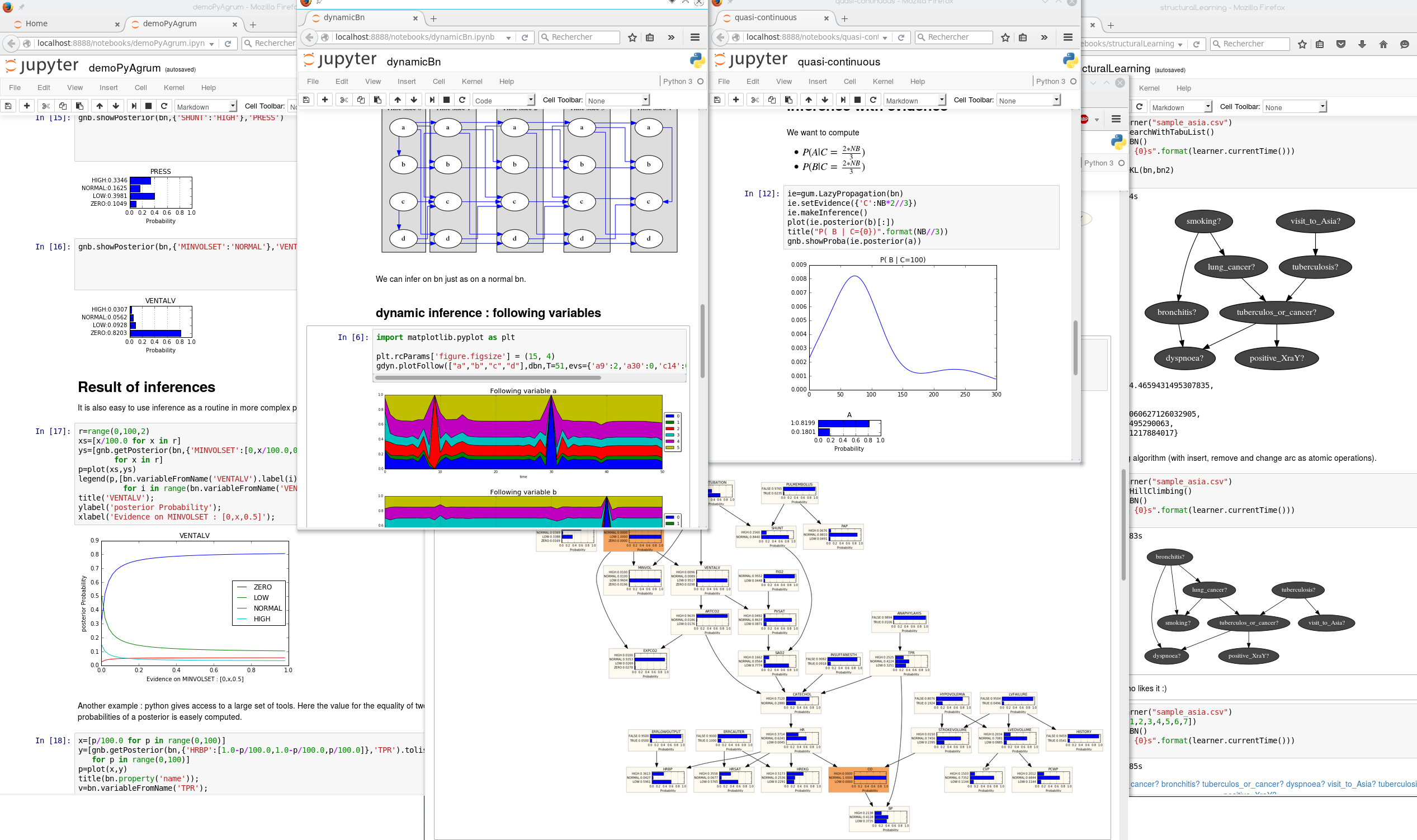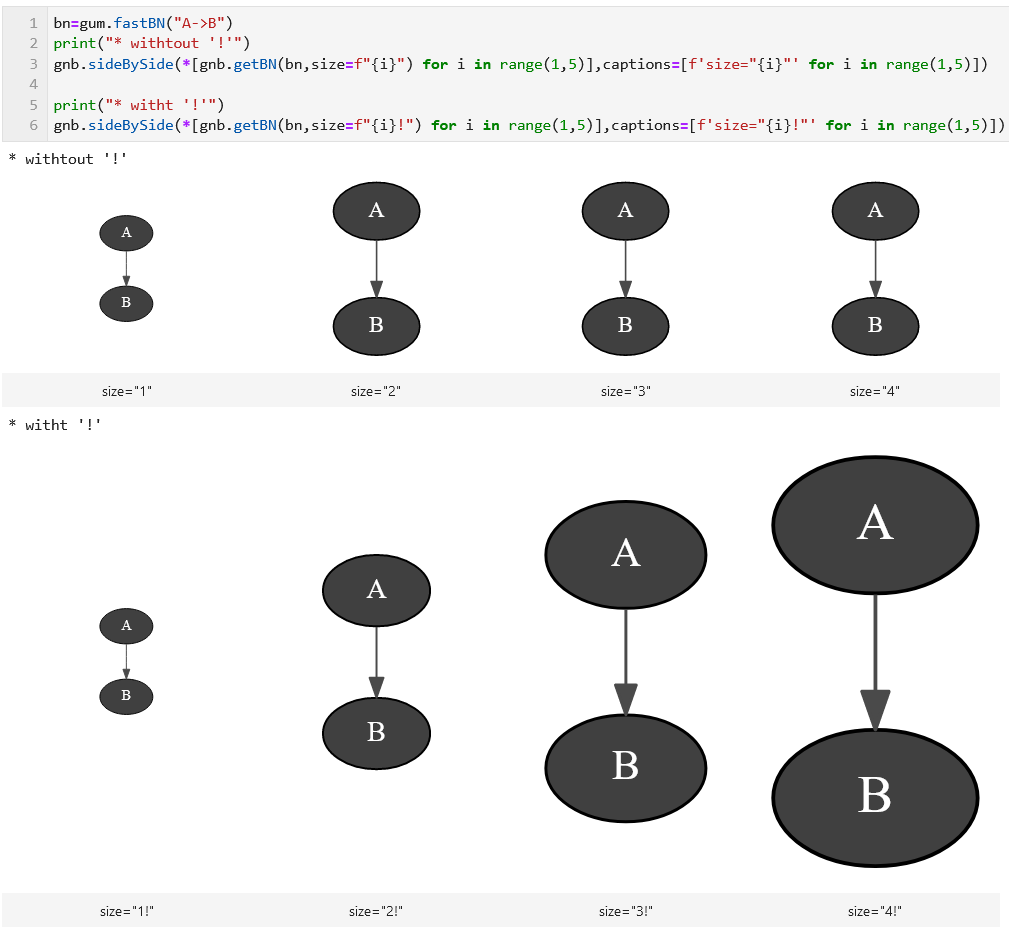# pyAgrum.lib.notebook¶

`pyAgrum.lib.notebook` aims to facilitate the use of pyAgrum with jupyter notebook (or lab).## Visualization of graphical models¶

Important

For many graphical representations functions, the parameter size is directly transferred to graphviz. Hence, Its format is a string containing an int. However if size ends in an exclamation point “!” (such as size=”4!”), then size is taken to be the desired minimum size. In this case, if both dimensions of the drawing are less than size, the drawing is scaled up uniformly until at least one dimension equals its dimension in size.pyAgrum.lib.notebook.showBN(bn, size=None, nodeColor=None, arcWidth=None, arcColor=None, cmap=None, cmapArc=None)

show a Bayesian network

Parameters
• bn – the Bayesian network

• size – size of the rendered graph

• nodeColor – a nodeMap of values (between 0 and 1) to be shown as color of nodes (with special colors for 0 and 1)

• arcWidth – a arcMap of values to be shown as width of arcs

• arcColor – a arcMap of values (between 0 and 1) to be shown as color of arcs

• cmap – color map to show the colors

• cmapArc – color map to show the arc color if distinction is needed

Returns

the graph

pyAgrum.lib.notebook.getBN(bn, size=None, nodeColor=None, arcWidth=None, arcColor=None, cmap=None, cmapArc=None)

get a HTML string for a Bayesian network

Parameters
• bn – the Bayesian network

• size – size of the rendered graph

• nodeColor – a nodeMap of values (between 0 and 1) to be shown as color of nodes (with special colors for 0 and 1)

• arcWidth – a arcMap of values to be shown as width of arcs

• arcColor – a arcMap of values (between 0 and 1) to be shown as color of arcs

• cmap – color map to show the colors

• cmapArc – color map to show the arc color if distinction is needed

Returns

the graph

pyAgrum.lib.notebook.showInfluenceDiagram(diag, size=None)

show an influence diagram as a graph

Parameters
• diag – the influence diagram

• size – size of the rendered graph

Returns

the representation of the influence diagram

pyAgrum.lib.notebook.getInfluenceDiagram(diag, size=None)

get a HTML string for an influence diagram as a graph

Parameters
• diag – the influence diagram

• size – size of the rendered graph

Returns

the HTML representation of the influence diagram

pyAgrum.lib.notebook.showMN(mn, view=None, size=None, nodeColor=None, factorColor=None, edgeWidth=None, edgeColor=None, cmap=None, cmapEdge=None)

show a Markov network

Parameters
• mn – the markov network

• view – ‘graph’ | ‘factorgraph’ | None (default)

• size – size of the rendered graph

• nodeColor – a nodeMap of values (between 0 and 1) to be shown as color of nodes (with special colors for 0 and 1)

• factorColor – a function returning a value (beeween 0 and 1) to be shown as a color of factor. (used when view=’factorgraph’)

• edgeWidth – a edgeMap of values to be shown as width of edges (used when view=’graph’)

• edgeColor – a edgeMap of values (between 0 and 1) to be shown as color of edges (used when view=’graph’)

• cmap – color map to show the colors

• cmapEdge – color map to show the edge color if distinction is needed

Returns

the graph

pyAgrum.lib.notebook.getMN(mn, view=None, size=None, nodeColor=None, factorColor=None, edgeWidth=None, edgeColor=None, cmap=None, cmapEdge=None)

get an HTML string for a Markov network

Parameters
• mn – the markov network

• view – ‘graph’ | ‘factorgraph’ | None (default)

• size – size of the rendered graph

• nodeColor – a nodeMap of values (between 0 and 1) to be shown as color of nodes (with special colors for 0 and 1)

• factorColor – a function returning a value (beeween 0 and 1) to be shown as a color of factor. (used when view=’factorgraph’)

• edgeWidth – a edgeMap of values to be shown as width of edges (used when view=’graph’)

• edgeColor – a edgeMap of values (between 0 and 1) to be shown as color of edges (used when view=’graph’)

• cmap – color map to show the colors

• cmapEdge – color map to show the edge color if distinction is needed

Returns

the graph

pyAgrum.lib.notebook.showCN(cn, size=None, nodeColor=None, arcWidth=None, arcColor=None, cmap=None, cmapArc=None)

show a credal network

Parameters
• cn – the credal network

• size – size of the rendered graph

• nodeColor – a nodeMap of values (between 0 and 1) to be shown as color of nodes (with special colors for 0 and 1)

• arcWidth – a arcMap of values to be shown as width of arcs

• arcColor – a arcMap of values (between 0 and 1) to be shown as color of arcs

• cmap – color map to show the colors

• cmapArc – color map to show the arc color if distinction is needed

Returns

the graph

pyAgrum.lib.notebook.getCN(cn, size=None, nodeColor=None, arcWidth=None, arcColor=None, cmap=None, cmapArc=None)

get a HTML string for a credal network

Parameters
• cn – the credal network

• size – size of the rendered graph

• nodeColor – a nodeMap of values (between 0 and 1) to be shown as color of nodes (with special colors for 0 and 1)

• arcWidth – a arcMap of values to be shown as width of arcs

• arcColor – a arcMap of values (between 0 and 1) to be shown as color of arcs

• cmap – color map to show the colors

• cmapArc – color map to show the arc color if distinction is needed

Returns

the graph

pyAgrum.lib.notebook.showInference(model, **kwargs)

show pydot graph for an inference in a notebook

Parameters
• model (GraphicalModel) – the model in which to infer (pyAgrum.BayesNet, pyAgrum.MarkovNet or pyAgrum.InfluenceDiagram)

• engine (gum.Inference) – inference algorithm used. If None, gum.LazyPropagation will be used for BayesNet, gum.ShaferShenoy for gum.MarkovNet and gum.ShaferShenoyLIMIDInference for gum.InfluenceDiagram.

• evs (dictionnary) – map of evidence

• targets (set) – set of targets

• size (string) – size of the rendered graph

• nodeColor – a nodeMap of values (between 0 and 1) to be shown as color of nodes (with special colors for 0 and 1)

• factorColor – a nodeMap of values (between 0 and 1) to be shown as color of factors (in MarkovNet representation)

• arcWidth – a arcMap of values to be shown as width of arcs

• arcColor – a arcMap of values (between 0 and 1) to be shown as color of arcs

• cmap – color map to show the color of nodes and arcs

• cmapArc – color map to show the vals of Arcs.

• graph – only shows nodes that have their id in the graph (and not in the whole BN)

• view – graph | factorgraph | None (default) for Markov network

Returns

the desired representation of the inference

pyAgrum.lib.notebook.getInference(model, **kwargs)

get a HTML string for an inference in a notebook

Parameters
• model (GraphicalModel) – the model in which to infer (pyAgrum.BayesNet, pyAgrum.MarkovNet or pyAgrum.InfluenceDiagram)

• engine (gum.Inference) – inference algorithm used. If None, gum.LazyPropagation will be used for BayesNet, gum.ShaferShenoy for gum.MarkovNet and gum.ShaferShenoyLIMIDInference for gum.InfluenceDiagram.

• evs (dictionnary) – map of evidence

• targets (set) – set of targets

• size (string) – size of the rendered graph

• nodeColor – a nodeMap of values (between 0 and 1) to be shown as color of nodes (with special colors for 0 and 1)

• factorColor – a nodeMap of values (between 0 and 1) to be shown as color of factors (in MarkovNet representation)

• arcWidth – a arcMap of values to be shown as width of arcs

• arcColor – a arcMap of values (between 0 and 1) to be shown as color of arcs

• cmap – color map to show the color of nodes and arcs

• cmapArc – color map to show the vals of Arcs.

• graph – only shows nodes that have their id in the graph (and not in the whole BN)

• view – graph | factorgraph | None (default) for Markov network

Returns

the desired representation of the inference

pyAgrum.lib.notebook.showJunctionTree(bn, withNames=True, size=None)

Show a junction tree of a Bayesian network

Parameters
• bn (pyAgrum.BayesNet) – the model

• withNames (bool) – names or id in the graph (names can created very large nodes)

• size (float|str) – size of the rendered graph

pyAgrum.lib.notebook.getJunctionTree(bn, withNames=True, size=None)

get a HTML string for a junction tree (more specifically a join tree)

Parameters
• bn – the Bayesian network

• withNames (boolean) – display the variable names or the node id in the clique

• size – size of the rendered graph

Returns

the HTML representation of the graph

## Visualization of Potentials¶

pyAgrum.lib.notebook.showProba(p, scale=1.0)

Show a mono-dim Potential (a marginal)

Parameters
pyAgrum.lib.notebook.getPosterior(bn, evs, target)

shortcut for proba2histo(gum.getPosterior(bn,evs,target))

Parameters
• bn (gum.BayesNet) – the BayesNet

• evs (dict(str->int)) – map of evidence

• target (str) – name of target variable

Returns

the matplotlib graph

pyAgrum.lib.notebook.showPosterior(bn, evs, target)

shortcut for showProba(gum.getPosterior(bn,evs,target))

Parameters
• bn – the BayesNet

• evs – map of evidence

• target – name of target variable

pyAgrum.lib.notebook.getPotential(pot, digits=None, withColors=None, varnames=None)

return a HTML string of a gum.Potential as a HTML table. The first dimension is special (horizontal) due to the representation of conditional probability table

Parameters
• pot (gum.Potential) – the potential to show

• digits (int) – number of digits to show

• withColors (bool) – bgcolor for proba cells or not

• varnames (List[str]) – the aliases for variables name in the table

Returns

the html representation of the Potential (as a string)

Return type

str

pyAgrum.lib.notebook.showPotential(pot, digits=None, withColors=None, varnames=None)

show a gum.Potential as a HTML table. The first dimension is special (horizontal) due to the representation of conditional probability table

Parameters
• pot (gum.Potential) – the potential to show

• digits (int) – number of digits to show

• withColors (bool) – bgcolor for proba cells or not

• varnames (List[str]) – the aliases for variables name in the table

## Visualization of graphs¶

pyAgrum.lib.notebook.getDot(dotstring, size=None)

get an HTML representation of a dot string

Parameters
• dotstring (str) – the dot string

• size (float|str) – size of the rendered graph

Return type

the HTML representation of the dot string

pyAgrum.lib.notebook.showDot(dotstring, size=None)

show a dot string as a graph

Parameters
• dotstring (str) – the dot string

• size (float|str) – size of the rendered graph

pyAgrum.lib.notebook.getGraph(gr, size=None)

get an HTML representation of a pydot graph

Parameters
• gr (pydot.Dot) – the graph

• size (float|str) – the size of the rendered graph

Return type

the HTML representation of the graph (as a string)

pyAgrum.lib.notebook.showGraph(gr, size=None)

show a pydot graph in a notebook

Parameters
• gr (pydot.Dot) – the graph

• size (float|str) – the size of the rendered graph

## Visualization of approximation algorithm¶

pyAgrum.lib.notebook.animApproximationScheme(apsc, scale=<ufunc 'log10'>)

show an animated version of an approximation algorithm

Parameters
• apsc – the approximation algorithm

• scale – a function to apply to the figure

## Helpers¶

pyAgrum.lib.notebook.configuration()

Display the collection of dependance and versions

pyAgrum.lib.notebook.sideBySide(*args, **kwargs)

display side by side args as HMTL fragment (using string, _repr_html_() or str())

Parameters
• args – HMTL fragments as string arg, arg._repr_html_() or str(arg)

• captions – list of strings (captions)